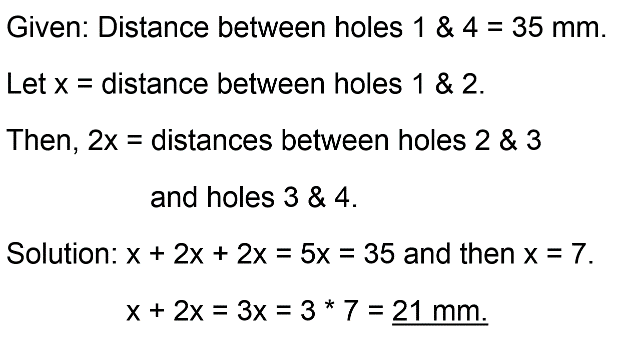Select Page

Each month, a new set of puzzles will be posted.  Come back next month for the solutions and a new set of puzzles, or subscribe to have them sent directly to you.

## MIND-Xpander puzzle

You have a piece of paper, 10 cm. by 10 cm. Area = 100 cm². For some reason, you need a square piece of paper with an area of 50 cm². Using the piece of paper, you have, what’s an easy way of getting the new square?

## Algebraic Challenge puzzle

By replacing each question mark with an algebraic sign (+, -, x and /), solve the following equations for the answers as shown:

(6 ? 4) ? 12 = 12

(7 ? 3) ? (4 ? 1) = 26

(4 ? 2) ? (4 ? 3) = 24

(6 ? 5) ? (9 ? 2) = 19

## Chinese Multiplication puzzle

Also known as a lattice method, the Chinese method provides an easy way to multiply any two numbers without the aid of a calculator. In the following puzzles, some or part of the multiplied or added values are provided. The object of this puzzle is to find the missing values to determine the original numbers that when multiplied together gives you the correct product as shown on the bottom line. Find these missing values.## Feedback

There are more than one way of doing these puzzles and may well be more than one answer.  Please let me and others know what alternatives you find by commenting below.  We also welcome general comments on the subject and any feedback you'd like to give.

If you have a question that needs a response from me or you would like to contact me privately, please use the contact form.

## Get more puzzles!

If you've enjoyed doing the puzzles, consider ordering the books;

• Book One - 150+ of the best puzzles
• Book Two - 200+ with new originals and more of your favourites

Both in a handy pocket sized format.   Click here for full details.

## MIND-Xpander Logic Problem

Four holes are drilled in a straight line in a rectangular steel plate. The distance between hole 1 and 4 is 35 mm. The distance between hole 2 and hole 3 is twice the distance between hole 1 and hole 2. The distance between hole 3 and hole 4 is the same as the distance between hole 2 and hole 3. What is the distance in milli-metres, between hole and hole 3?

Solution## Can-U-Figure-It-Out Puzzles

How many squares, of any size, are there in the following diagram?

Solution## EQUATE+0 Puzzle

Each row, column & diagonal is an equation and you use the numbers 1 to 9 to complete the equations. Each number can be used only once. ‘No’ numbers have been provided to get you started. Find the remaining seven numbers that satisfies all the resulting equations. Note – multiplication (x) & division (/) are performed before addition (+) and subtraction (-).

Solution